BFOIT - Introduction to Computer Programming

## Lastly## Introduction

Many lessons ago, you learned a way to repeat a list of instructions some number of times.  This allowed us to reduce the size of our programs.  Our programs were thus easier to enter correctly, easier to read, and easier to modify.  We used repeat when we noticed we were doing something identical over and over.

A few lessons later we learned how to write procedures with inputs.  Procedures with inputs allowed us to do the same sort of thing, with differences specified by the inputs.  As an example, we could write a procedure that draws a box that is any size.  The size of a particular box is specified when the box procedure is invoked.

Successful use of these features of a programming language depend upon a programmer's ability to notice patterns in the code they have written or are thinking about writing.  This is what makes programming fun and a bit challenging - the programmer is constantly solving puzzles.

In this lesson you will be challenged to discover patterns that are a bit more subtle.  And, the structure of the procedures that you write will also be a bit more complex.  But, I've got some fun programs for you to write, puzzles for you to solve.

In this lesson, you will learn

1. A more powerful way to iterate some set of instructions.  You are going to invoke a procedure from within its own definition.  This is called recursion.
2. How to stop execution of a procedure without outputing a value.

## Drawing a Squiral

Take a close look at the trail the turtle is making in Figure 13.1.Figure 13.1

What are the instructions that can be given to get the turtle to draw this squareish spiral?  Here is the TG applet; play around a bit until you get it.  Make sure to try for a bit before you read on!

What you need to do is move the turtle forward, turn 90 degrees, go forward a little bit further than you did last time, turn 90 degrees, go forward - again, a little bit further than last time, etc...

Do you see the pattern in what we are instructing the turtle to do?

Here are the instructions lined up to make the comparison easier.

 `````` forward 5 right 90 forward 10 right 90    forward 15 right 90 forward 20 right 90 forward 25 right 90 ... ``````

There is an obvious pattern.  But, the repeat command will not work because the instructions in the pattern are not identical - the number of steps the turtle takes forward keeps increasing.  We need a way to change the input to the forward command.  This is the exact need that I described in the introduction for the lesson on adding inputs to procedures which you write.

So...  Can we use procedures with inputs to iterate through similar instructions, like those above?

Yes!  And it's really cool how we do it.  Read on...

## Recursion

So far...

• when you want to repeat doing some instructions a number of times (iteration), you put what you want to do inside the square brackets of a repeat instruction.
• when you want to do things that are sort-of the same, but different in ways that can be handled with parameters, you put what you want to do in a procedure that has inputs for the things that are different, the parameters.

Recursion, which is invoking a procedure from within the body of the procedure, is the combination of these two important programming concepts.

With recursion you get the iteration of some instructions with the flexibility provided by a procedure with inputs that affect what happens.

Here's a start at our squiral program.

 `````` to squiral :steps forward :steps right 90    squiral sum :steps 5 end ``````

Looks simple enough, doesn't it?  Go back up to the TG applet; open the Editor window and type in this definition.  Then, invoke squiral with an input of 5 in the CommandCenter.

 `` squiral 5    ``

What happens?

If you didn't make a typing error, you got the square spiral we wanted.

But, if you try to get the turtle to do something else, you'll notice that it isn't listening to you.  It's still BUSY.  You might not have ever noticed this before - checkout the rightmost side of the CommandCenter name stripe.Figure 13.2

The problem is that squiral will never complete.  It is easy to get into this situation when you are learning about recursion.

So, how do you get out of this situation?  Hold down the [Ctrl] key and press Q (which stands for QUIT).  For this to work, the CommandCenter must be the active subwindow, the one that has keyboard focus.  You can tell by looking at its namestripe; it should be black and not gray, and its cursor should be blinking.  When typing [Ctrl]-Q works, the "BUSY" tag in the CommandCenter's namestripe disappears.

Now - back to squiral.

What we need is called a stop rule.  A stop rule is an instruction that terminates the recursive execution.

You already know one way of terminating the execution of a procedure: OUTPUT.  However, if a procedure OUTPUTs a value, it has to go somewhere.  So, there's another command, STOP, which terminates execution of the current procedure - without producing a value.

Here's are descriptions of OUTPUT and STOP.

 Name Input Description OUTPUT value Execution of the current procedure is complete.  OUTPUT's input (value) is passed back to the instruction that the current procedure's invocation is part of. STOP The current invocation of the procedure in which STOP exists terminates.

We can use an IF instruction with STOP to force our squiral program to terminate.  Here's how.  Try it out in the TG applet above.

 `````` to squiral :steps if greater? :steps 50 [stop]    forward :steps right 90 squiral sum :steps 5 end ``````

In this example, I picked the ending value of 50 for a specific reason.  Once you have squiral working and before you increase this ending value, I want you to trace the execution of squiral.  If you don't remember how to use trace, I introduced it in the lesson: "Defining Your Own Operators".

So, use trace to see what is going on when you execute a recursive procedure.  In this case, you are not using trace to help with debugging; you are using it to give you a visualization of a pattern produced in executing a recursive procedure.

## Project: Drawing a Tree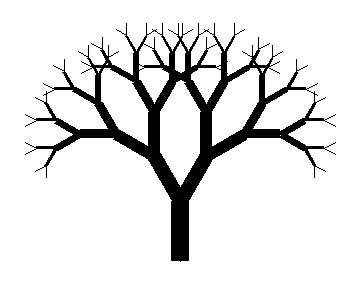Figure 13.3

Alright, what is the pattern in this tree?  What is the minimum object that can be drawn over and over that results in a tree that's at least similar to this one?

Well, the first thing I did when I wrote my version of the program was to simplify the problem.  Even though I noticed that the branches of the tree get thinner the further out from the trunk, I decided to skip this detail at first.  My style of programming is to get something working first, and then add complexity.  So, Figure 13.4 shows a tree with a constant pen size of 1.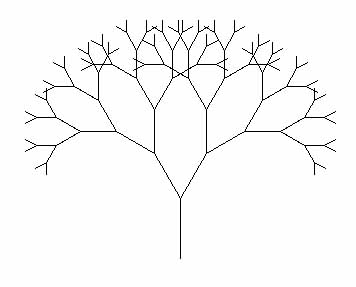Figure 13.4

Look close - the tree is nothing but a bunch of 'Y's.  In Figure 11.5, I've highlighted the 'Y's making up the leftmost edge of the tree.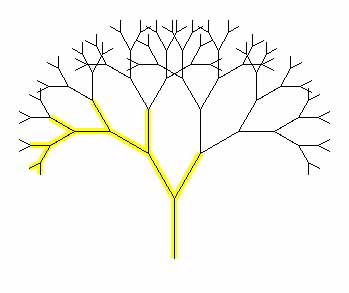Figure 13.5

The biggest clue you've got on how to solve this puzzle is that the solution has to be recursive, otherwise I wouldn't have included it in this lesson.  And, looking at the tree with the highlighting, it's obvious that we need to write a procedure that draws a 'Y'.

I'll give you the code for a 'Y' so that we can concentrate on the main topic, recursion.  Type the following procedure definition into TG's Editor window.

 `````` to tree :steps forward :steps left 30 forward difference :steps 8 back difference :steps 8    right 60 forward difference :steps 8 back difference :steps 8 left 30 back :steps end ``````

See what tree does.  Type in an invocation of it in the CommandCenter window.

 `` tree 60   ``

OK we have a 'Y'.  Next, the best guess is that the lines of instructions that draw the branches need to be replaced with invocations of tree.  Right?  It's sort of like squiral except that the 'Y's get smaller.

 `````` to tree :steps forward :steps left 30 tree difference :steps 8    right 60 tree difference :steps 8 left 30 back :steps end ``````

What's missing?  Test it out.

The tree starts out looking like the leftmost lines in Figure 13-5.  But then the turtle keeps on drawing, what seems to look like some sort of spiral!

It's missing a stop rule.  Since each recursive invocation of tree decreases the input steps, we can use this to determine when the procedure should just STOP.

 `````` to tree :steps if less? :steps 5 [stop] forward :steps left 30 tree difference :steps 8    right 60 tree difference :steps 8 left 30 back :steps end ``````

OK.  This works...  Now your turn to complete the project.  Add code that decreases the width of the branches of the tree.  As the branches become smaller in length, decrease their width.

## The von Koch Curve and Snowflake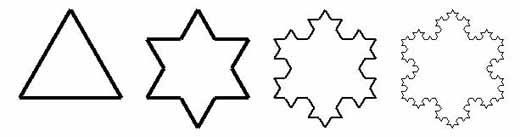Figure 13.6

A Koch SnowFlake, first described by Helge von Koch in 1904, is one kind of a thing called a fractal.  To draw one, you start with an equilateral triangle (see the left-most object in Figure 13.6).  For each side of the triangle, you

1. divide it into three equal parts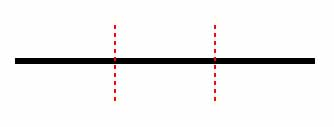Figure 13.7
2. replace the inner third of it with an equilateral triangle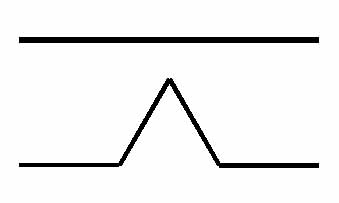Figure 13.8
3. repeat the first two steps on all lines of the new figure - this can be done indefinitelyFigure 13.9

This is another classic problem where recursion saves the day.  Have fun.  Treat this as a puzzle.  Don't be afraid to just play around at first.  Get something working and then refine your program until you have an elegant solution...

If you've given this little puzzle a good attempt but seem to have reached a dead end, here is some help.

## Sierpinski Triangle

Here is another bit of artwork that is commonly created with recursion.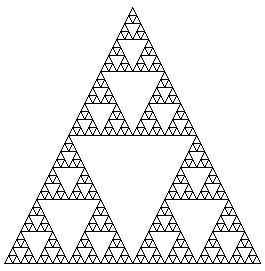Figure 13.10

The triangle forming the perimeter, the leftmost drawing in Figure 13.11, is not part of the recursive process.  The second step consists of placing an upside-down triangle in the center of the existing triangle.  Doing this creates three new triangles, one above, one to the left, and one to the right.  Now, the recursive process starts.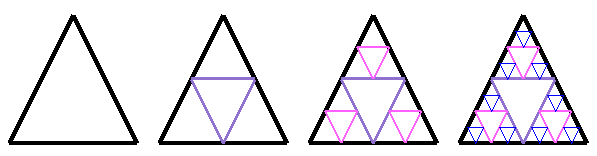Figure 13.11

The programming language you learn first can really effect (?limit?) how you approach writing programs.  It is no accident that I have introduced recursion before the advanced form of REPEAT.  The little projects that we have done so far in this lesson were carefully chosen in that they are best solved in an iterative manner with recursion.  What the procedures consisted of was something performed that was a subset of the whole solution.  The recursive invocation changed the input(s) in some manner to move it/them closer towards the condition tested by the stop rule.

Look back at our finished squiral program. It first checks to see if the number of steps the turtle is being asked to move forward is more than the desired maximum.  If so, we're done.  If not, the turtle is instructed to more forward and then turn to the right.  Finally, in the recursive invovation, we increase the number of steps the turtle will move next time.  This is a very straight-forward description of what we want done.

But, there is another way to get the turtle to draw a squiral.  And, sadly in my opinion, it's often the way students are taught to write squiral.  The number of steps the turtle is requested to move forward can be expressed as a mathematical formula.  In this case, it's a pretty easy one to see,

 `` steps = 5 * forwardMoveNumber ``

where forwardMoveNumber is 1 the first time the turtle moves forward, 2 the second time, 3 the third time, etc...  Think about this series of numbers.  It is the same set as the one the REPEAT command could be using as it counts the number of times it has performed its instruction list.

The REPEAT command is more powerful than I've so far explained.  You do do have access to the counter that does exist - that REPEAT maintains to know when it's done.  You access the counter via the REPCOUNT operator.  Checkout the following example in Figure 13.12, then try it out for yourself in the TG applet above.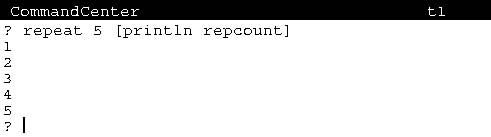Figure 13.12

So, what good is this?

Well, you could have written the squiral program using repcount without recursion.  Checkout the following code; study it, figure out how it works, and finally try it out...

 `````` to squiral :maxSteps repeat (quotient :maxSteps 5) [forward (product repcount 5) right 90]    end ``````

Invoke it from the CommandCenter with some input, .e.g., 200.

 `` squiral 200  ``

But, remember that writing correct programs is always going to be you biggest challenge.  So I personally believe that the recursive version of squiral is easier to understand.  It basically tells the turtle to keep drawing lines that are 5 steps longer (after turning to the right) until it has walked some maximum number of steps.  The version using REPEAT and REPCOUNT is based on a couple of arithmetic expressions which you need to figure out before you really see what's going on...

So, when is using REPEAT with REPCOUNT good?  Where does it make sense to use it?

## Project: Graphing Equations

Graphing calculators are popular these days.  But, now that you know Logo and have TG, you can instruct the turtle to graph ANY equation you want.

Checkout the line drawn in Figure 13.13; it is the results of plotting the equation: y = x/2 + 20.

 y = x/2 + 20Figure 13.13

You know how to draw a point; we did this way back when we instructed the turtle to draw a circle by drawing lots of points.  Here's one way, assuming a pensize of 4.

 `````` to drawPoint pendown forward 2 back 4 forward 2    end ``````

Chances are that you learned how to draw a straight line via the slope-intercept method in Algebra class.  If you didn't or you want to refresh your memory, here is a tutorial on it.

Given this, here is the rest of the code for drawing the line.  Combine this with the axes you drew at the end of lesson 5 to get something similar to Figure 13.13.

 `````` to black output 0 end to blue output 1 end to north output 0 end to east output 90 end to gotoPoint :x :y setxy :x :y end to gotoNextPoint :rise :run setheading north forward :rise setheading east forward :run end ;draw points on a line via slope-intercept method to drawLine :yIntercept :rise :run penup gotoPoint 0 :yIntercept repeat 150 [drawPoint penup gotoNextPoint :rise :run] penup gotoPoint 0 :yIntercept repeat 150 [drawPoint penup gotoNextPoint minus :rise minus :run]    end ;for equation: y = x/2 + 20 ;the y-intercept=20, rise=1, run=2 to graphEquation setpencolor blue drawLine 20 1 2 end to main home clean hideturtle setpencolor black ;axes graphEquation end ``````

But, this method draws a lousy line if the slope is either very shallow or very steep.  Type in the code I've given you.  Get it to work, then modify it to plot the equation: y = x/10 + 50.  See what I mean?

This is where an advanced form of REPEAT can make this task trivial.   And, it will also allow you to draw lines for equations that the slope-intercept method doesn't handle, e.g., y = x2 - 7.

The definition for line gives us a clue on how to approach the problem.  Here is a definition for line that I found on the Internet.

```
A line is a spatial feature... that can be described by a series of coordinate pairs.
```

So to draw a line, all we need to do is place the turtle on one of the end points of a line segment to be drawn (a coordinate pair), put the pen down, and then move it to a series of consecutive points.  The points (coordinate pairs) can be produced by solving the concerned equation for a series of values.

For the equation y = x2 - 7, we can write a procedure that gives us the Y coordinate for an X coordinate provided as an input.  Here it is.

 `````` to yValue :xValue output difference (product :xValue :xValue) 7    end ``````

Given this, we can get the turtle to move to any point on the line with the procedure gotoPoint that was defined above.  Now all we need is a way of iterating through a series of X coordinate values.  REPEAT and REPCOUNT almost get us there - the only gotcha is that so far all we know how to do is access a count that goes from 1 to some larger positive number.  To draw a line representing an equation, we need access to negative values for X coordinates.

### REPEAT With Start, End, and Increment Number Values

As of version .9.23 of TG, the REPEAT command can do this for you.  The first input to REPEAT can be a sentence consisting of three numbers,

1. a starting number,
2. an ending number, and
3. an increment value.

Figure 13.14 shows an example.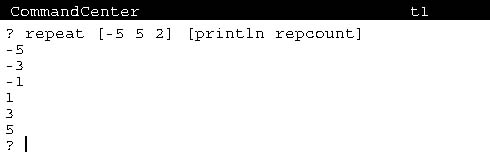Figure 13.14

So, with this advanced form of REPEAT, a series of X coordinate values can be generated.  Here is a new version of a complete program including a new drawLine that uses REPEAT.

 `````` to gotoPoint :x :y setxy :x :y end ; given an X value ; output a coresponding Y value for the equation: y = x2 - 7    to yValue :xValue output difference (product :xValue :xValue) 7 end to drawLine penup gotoPoint -300 (yValue -300) pendown repeat [-300 300 5] [setxy repcount (yValue repcount)] end to main home clean drawLine end ``````

There you have it!  I've used REPEAT and REPCOUNT to iterate through a series of X coordinates, computing corresponding Y coordinates, and using setxy to move the turtle through the path of the points.

### Graphing With Scaling

Figure 13.15 shows the graph drawn by my finished program.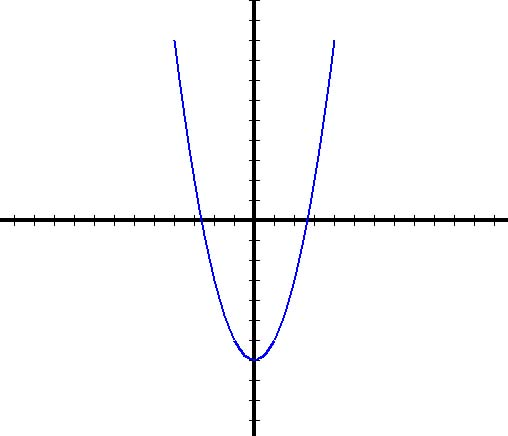Figure 13.15

It is a little different from what you see given the program above.  This is because Y values get very large.  You can see this by using trace.  In the CommandCenter, enter "trace yValue" and then run the program again.  Figure 13.16 is the tailend of what was printed when I did this.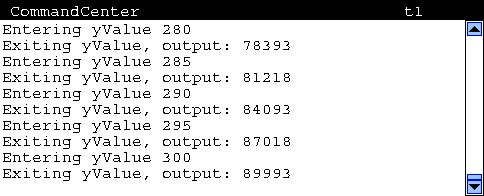Figure 13.16

To get your graph to look like mine, you need to scale the points.

What do I mean by scale the points?  Well, I have made twenty (20) turtleSteps equal to one (1) unit on the graph.  You must be familiar with scaling.  Maps have scales.  Models of things have scales.  In Figure 13.15, each of the tic marks on the axes are at 20 turtlestep increments, which means they are drawn at integer values.  So, the line you see is for a series of points starting with x = -4 and going through x = 4.

I'm leaving you with the challenge of writing a program that duplicates what my graph looks like.  So, you too need to add scaling to your program.

## Summary

I've given you an introduction to one of the most powerful mechanisms for writing software that you will ever learn.  Although recursion is often initially confusing and appears to work as if by magic, it actually only consists of a couple of simple pieces.

1. Recursion's base is the procedure with inputs.  The body of the procedure contains a recursive invocation, an invocation to itself.
2. The recursive invocation changes at least one of the inputs, which will change its behavior and move the process one step closer to terminating.
3. A stop rule must exist, consisting of an if command which tests whether an input matches the termination condition.

In addition to recursion, you learned advanced REPEAT command options.  You learned that while a REPEAT command is being performed, an operator named REPCOUNT outputs the current value of a variable that is being used to control when iteration is complete.  The values of this variable by default are 1 through the specified number of repetitions.  But, a REPEAT command may include a sentence composed of three numbers which control values of this variable - an initial value, a maximum value, and an increment.

Back to Predicates